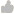> Knowledge base> Article: How do I convert MSI to Square Meters or Square Meters to MSI? How do I calculate MSI?

# How do I convert MSI to Square Meters or Square Meters to MSI? How do I calculate MSI?

Article ID: 44934Question
How do I convert MSI to Square Meters or Square Meters to MSI? How do I calculate MSI?

How do I convert MSI to Square Meters or Square Meters to MSI? How do I calculate MSI?

Use the following formulas:

Convert Square Meters to MSI  - MSI = 1.5425 X m² (Square Meters)

Convert MSI to Square Meters - Square Meters = .64 X MSI

MSI = 1000 in²

How to calculate MSI:

Calculate how many total square inches are in the roll of stock.

Primera Stock is 1,250' long and 8.5" wide.

1250ft multiplied by 12in in a foot = 15000in

8.5in wide x 15000in long = 127,500 square inches

MSI = 1000 square inches

Divide the total square inches for your roll by 1000

127500 / 1000 = 127.5 MSI

To calculate the cost, take the amount you paid for the roll and divide that by how many MSI you have in the roll.

\$392.00 / 127.5 MSI = \$3.07\MSI

You would then take this cost per MSI and put it into the Status Monitor Cost Estimator.

related articles

Article Details
 Views: 3353 Created on: May 24, 2014 Date updated: Oct 06, 2016 Posted in: CX1200-FX1200-CX1000-FX1000

 Poor 12345 Outstanding